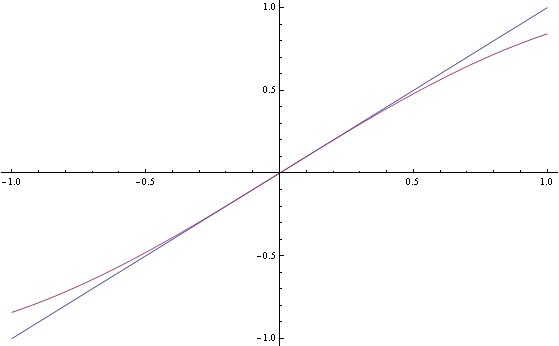# Why does differentiation find the approximate value?

Like imagine I have to find the cube root of 8.03. So I cube 8 and use the dy=8+dy/dx*(8.03-8.00) formula. But why is this finding the value of cube root of 8.03 and why is this value approximate instead of exact?

## Answers and Replies

What is the derivative at 8, conceptually?

1/12

Mark44
Mentor
Like imagine I have to find the cube root of 8.03. So I cube 8 and use the dy=8+dy/dx*(8.03-8.00) formula. But why is this finding the value of cube root of 8.03 and why is this value approximate instead of exact?
Look at the graph of ##y = x^{1/3}##. The derivative, dy/dx, gives the slope of the tangent line to this curve. The formula you show gives you the y values on the tangent line, which is close to, but slightly different from the y values on the curve.

Since the tangent line at (8, 2) lies above the curve ##y = x^{1/3}## , the approximate values will be a little larger than the values on the curve.

Mark44
Mentor
Number Nine said:
What is the derivative at 8, conceptually?
1/12
The value of the derivative isn't what Number Nine was asking. He was asking about the meaning of the derivative at that point.

Your replies made me realize all the holes in my calculus knowledge. I've been taught calculus in school just through formulas so it looks like my concepts are very weak. I have no idea what to do about it because my textbook also only uses formulas.

Mark44
Mentor
Your replies made me realize all the holes in my calculus knowledge. I've been taught calculus in school just through formulas so it looks like my concepts are very weak. I have no idea what to do about it because my textbook also only uses formulas.
Most calculus texts contain formulas, but they usually contain explanatory text as well. Are you saying that your textbook doesn't have explanations to go with the formulas? Sometimes students focus on the formulas and ignore the surrounding text.

Basically it comes from this really simple idea: "The tangent line to ##x_0## resembles the curve near ##x_0##". For instance the curve defined by ##y=\sin x## resembles the tangent line to it at ##0## near zero:For example it is really hard to determine ##\sin(0.1\,\rm rad)## without using a calculator. But since the tangent line has a simple form, namely ##y=mx+b##, one can easily exploit the fact that the tangent line resembles the curve of ##\sin x## near ##0## since ##0.1## is approximately zero to find a rough estimate for ##\sin(0.1\,\rm rad)##.

Most calculus texts contain formulas, but they usually contain explanatory text as well. Are you saying that your textbook doesn't have explanations to go with the formulas? Sometimes students focus on the formulas and ignore the surrounding text.

My textbook contains examples of HOW to use, but not WHY

Mark44
Mentor
My textbook contains examples of HOW to use, but not WHY
Assuming each section of your textbook ends with a set of problems, maybe these problems are the WHY the formulas are used.